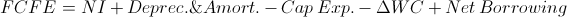# Free Cash Flow to Equity

FCFE Calculator (Click Here or Scroll Down)The formula for free cash flow to equity is net income minus capital expenditures minus change in working capital plus net borrowing.

The free cash flow to equity formula is used to calculate the equity available to shareholders after accounting for the expenses to continue operations and future capital needs for growth.

## Breakdown of FCFE Formula

Net Income is found on a firm's income statement and is the firm's earnings after expenses, including interest expenses and taxes. Net income may also be found on the cash flow statement which may save time considering other factors of the free cash flow to equity formula are on there as well. Net income may be referred to as "the bottom line".

A firm's prior capital expenditures can be found on its cash flow statement and represents capital used for long term or fixed assets.

A firm's working capital is current assets minus current liabilities. The term "current" implies that the assets and liabilities are liquid, generally representing less than one year, and used for short term operations. Current assets and current liabilities can be found on a firm's balance sheet.

Net borrowing is the difference between the amount a company borrows and what debt it repays. It is important to not include interest as this is already figured into net income (interest expense). Net borrowing can be found by comparing changes on a company's balance sheet.

## Use of the FCFE Formula

The free cash flow to equity formula may be used by investors and analysts in replace of dividends when analyzing a company. One of the most notable examples of this is in the free cash flow to equity model for valuing a stock. The free cash flow to equity model differs from the dividend discount method only in that it uses free cash flow to equity instead of dividends.

To understand the use the free cash flow to equity formula, one must understand the components of it and how it differs from dividends. A company's net income is also referred to its earnings. A company pays some of the earnings out to investors in the form of dividends and the amount retained is used for future growth. The formula for cash flow to equity starts with the company's earnings. Capital expenditures is substracted to account for the amount needed for assets used for growth. The next variable, change in working capital, is subtracted to account for an increase in capital needed for short term operations. Lastly, net borrowing is added, or subtracted if negative, to account for any capital received from taking new debt, or lost due to repayment of debt. These factors all resolve to the amount available to equity, or shareholders.

Although the free cash flow to equity may calculate the amount available to shareholders, it does not necessarily equate to the amount that is paid out to shareholders.

New to Finance?